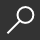## An Introduction to Classical Econometric Theory by Paul A. Ruud

Overview -
In An Introduction to Classical Econometric Theory Paul A. Ruud shows the practical value of an intuitive approach to econometrics. Students learn not only why but how things work. Through geometry, seemingly distinct ideas are presented as the result of one common principle, making econometrics more than mere recipes or special tricks. In doing this, the author relies on such concepts as the linear vector space, orthogonality, and distance. Parts I and II introduce the ordinary least squares fitting method and the classical linear regression model, separately rather than simultaneously as in other texts. Part III contains generalizations of the classical linear regression model and Part IV develops the latent variable models that distinguish econometrics from statistics. To motivate formal results in a chapter, the author begins with substantive empirical examples. Main results are followed by illustrative special cases; technical proofs appear toward the end of each chapter. Intended for a graduate audience, An Introduction to Classical Econometric Theory fills the gap between introductory and more advanced texts. It is the most conceptually complete text for graduate econometrics courses and will play a vital role in graduate instruction.

local_shippingFor DeliveryLimited Availability. Ships in 2-4 weeks.
This item is Non-Returnable.
FREE Shipping for Club Members helpNew & Used Marketplace 12 copies from \$72.40

## More About An Introduction to Classical Econometric Theory by Paul A. Ruud

### Overview

In An Introduction to Classical Econometric Theory Paul A. Ruud shows the practical value of an intuitive approach to econometrics. Students learn not only why but how things work. Through geometry, seemingly distinct ideas are presented as the result of one common principle, making econometrics more than mere recipes or special tricks. In doing this, the author relies on such concepts as the linear vector space, orthogonality, and distance. Parts I and II introduce the ordinary least squares fitting method and the classical linear regression model, separately rather than simultaneously as in other texts. Part III contains generalizations of the classical linear regression model and Part IV develops the latent variable models that distinguish econometrics from statistics. To motivate formal results in a chapter, the author begins with substantive empirical examples. Main results are followed by illustrative special cases; technical proofs appear toward the end of each chapter. Intended for a graduate audience, An Introduction to Classical Econometric Theory fills the gap between introductory and more advanced texts. It is the most conceptually complete text for graduate econometrics courses and will play a vital role in graduate instruction.

This item is Non-Returnable.

### Details

• ISBN-13: 9780195111644
• ISBN-10: 0195111648
• Publisher: Oxford University Press, USA
• Publish Date: March 2000
• Page Count: 976
• Dimensions: 9.63 x 7.92 x 1.67 inches
• Shipping Weight: 3.72 poundsRelated Categories